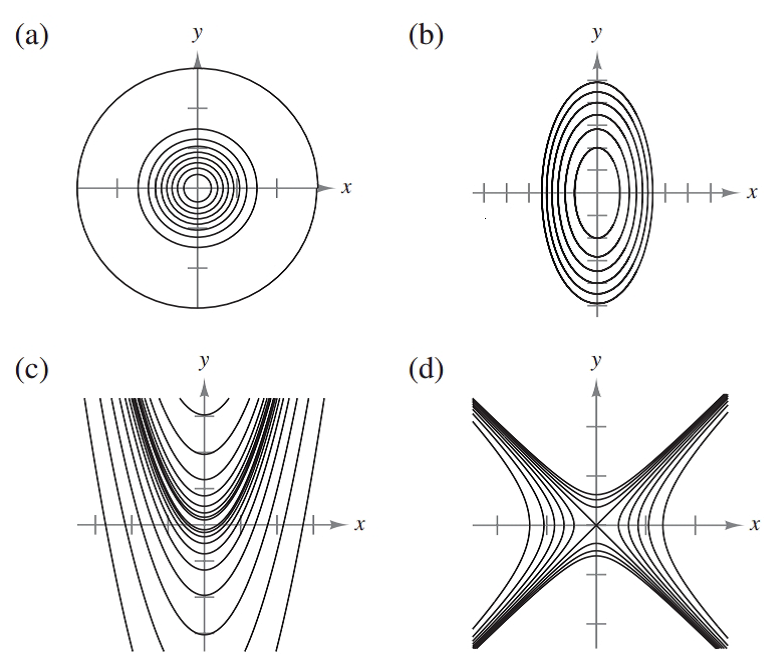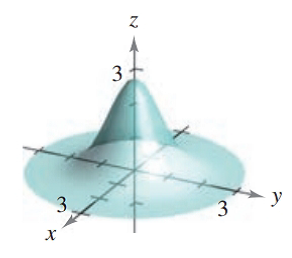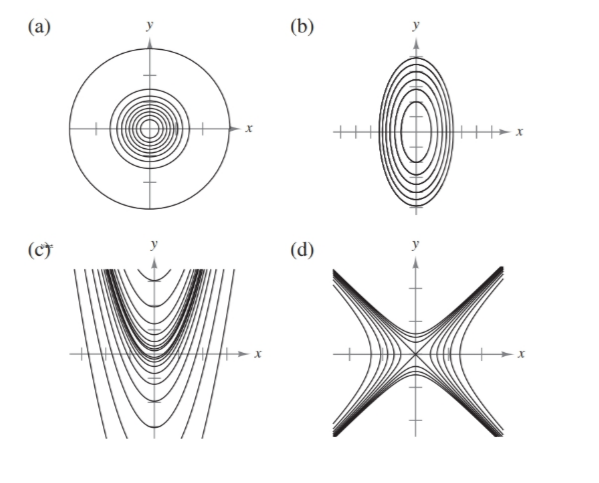Chapter 7.3, Problem 33E### Calculus: An Applied Approach (Min...

10th Edition
Ron Larson
ISBN: 9781305860919

#### Solutions

Chapter
Section### Calculus: An Applied Approach (Min...

10th Edition
Ron Larson
ISBN: 9781305860919
Textbook Problem
1 views

# Matching In Exercises 31-34, match the graph of the surface with one of the contour maps. [The contour maps are labeled (a)-(d).]f ( x , y ) = e 1 − x 2 − y 2To determine

The correct match for the function f(x,y)=e1x2y2 from the following options:Explanation

Given Information:

The option of provide function f(x,y)=e1x2y2 are:

The graph of equation f(x,y)=e1x2y2 given below,

Consider the provide function,

f(x,y)=e1x2y2

Substitute f(x,y)=C1 in the equation f(x,y)=e1x2y2 to obtain contour map,

C1=e1x2y2

Take log both sides,

lnC1=lne1x2y21x2y2=C11x2+y2=1C11

This equation represent the equation of circle.

Substitute f(x,y)=C2 in the equation f(x,y)=e1x2y2 to obtain contour map,

C2=e1x2y2

Take log both sides,

lnC2=lne1x2y21x2y2=C21x2+y2=1C21

This equation represent the equation of circle

### Still sussing out bartleby?

Check out a sample textbook solution.

See a sample solution

#### The Solution to Your Study Problems

Bartleby provides explanations to thousands of textbook problems written by our experts, many with advanced degrees!

Get Started

#### Find more solutions based on key concepts